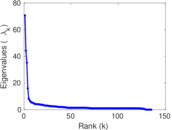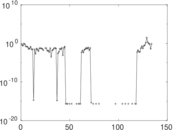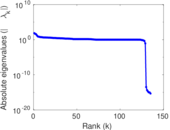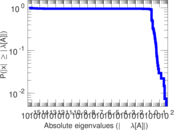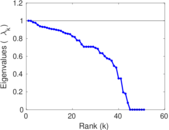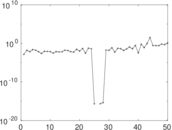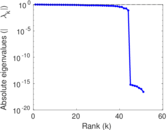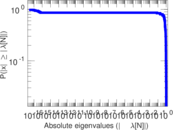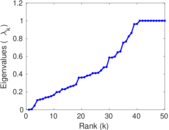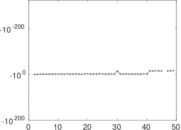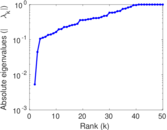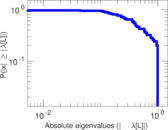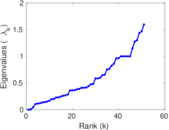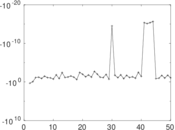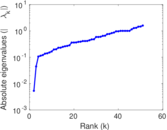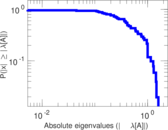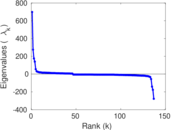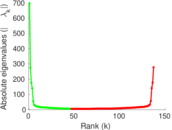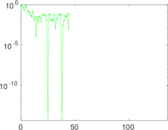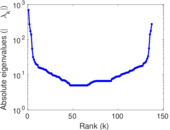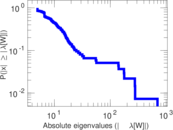# Wikibooks edits (ie)

This is the bipartite edit network of the Interlingue Wikibooks. It contains users and pages from the Interlingue Wikibooks, connected by edit events. Each edge represents an edit. The dataset includes the timestamp of each edit.

 Code `bie` Internal name `edit-iewikibooks` Name Wikibooks edits (ie) Data source http://dumps.wikimedia.org/ AvailabilityDataset is available for download Consistency checkDataset passed all tests Category Authorship network Dataset timestamp 2017-10-20 Node meaning User, article Edge meaning Edit Network formatBipartite, undirected Edge typeUnweighted, multiple edges Temporal dataEdges are annotated with timestamps

## Statistics

 Size n = 705 Left size n1 = 137 Right size n2 = 568 Volume m = 1,117 Unique edge count m̿ = 651 Wedge count s = 23,491 Claw count z = 1,192,943 Cross count x = 49,043,917 Square count q = 156 4-Tour count T4 = 96,978 Maximum degree dmax = 383 Maximum left degree d1max = 383 Maximum right degree d2max = 53 Average degree d = 3.168 79 Average left degree d1 = 8.153 28 Average right degree d2 = 1.966 55 Fill p = 0.008 365 89 Average edge multiplicity m̃ = 1.715 82 Size of LCC N = 431 Diameter δ = 12 50-Percentile effective diameter δ0.5 = 4.523 24 90-Percentile effective diameter δ0.9 = 7.422 94 Median distance δM = 5 Mean distance δm = 4.832 24 Gini coefficient G = 0.667 625 Balanced inequality ratio P = 0.235 452 Left balanced inequality ratio P1 = 0.174 575 Right balanced inequality ratio P2 = 0.332 140 Relative edge distribution entropy Her = 0.844 046 Power law exponent γ = 5.878 53 Tail power law exponent γt = 2.801 00 Tail power law exponent with p γ3 = 2.801 00 p-value p = 0.019 000 0 Left tail power law exponent with p γ3,1 = 2.171 00 Left p-value p1 = 0.180 000 Right tail power law exponent with p γ3,2 = 3.501 00 Right p-value p2 = 0.008 000 00 Degree assortativity ρ = −0.196 456 Degree assortativity p-value pρ = 4.367 48 × 10−7 Spectral norm α = 70.649 0 Algebraic connectivity a = 0.005 321 30 Spectral separation |λ1[A] / λ2[A]| = 1.598 66 Controllability C = 436 Relative controllability Cr = 0.627 338

## Plots

### Fruchterman–Reingold graph drawing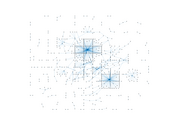### Degree distribution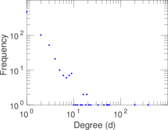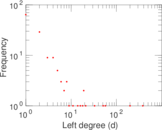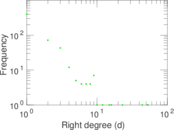### Cumulative degree distribution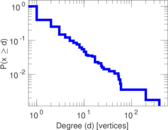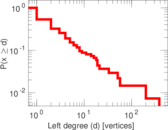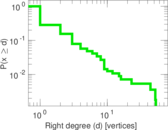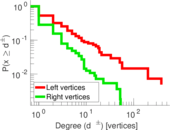### Lorenz curve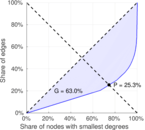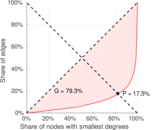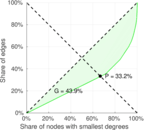### Spectral distribution of the adjacency matrix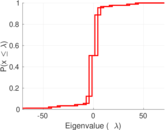### Spectral distribution of the normalized adjacency matrix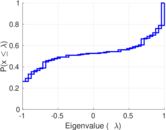### Spectral distribution of the Laplacian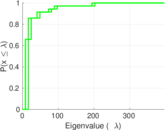### Spectral graph drawing based on the adjacency matrix### Spectral graph drawing based on the Laplacian### Spectral graph drawing based on the normalized adjacency matrix### Degree assortativity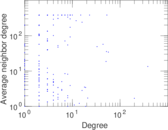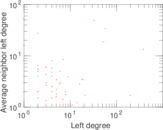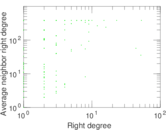### Zipf plot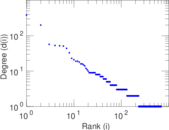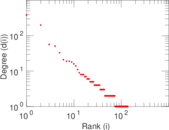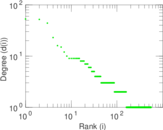### Hop distribution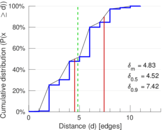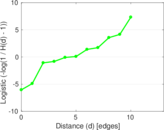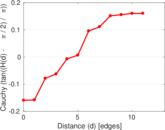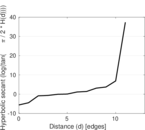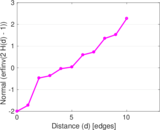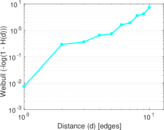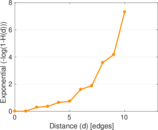### Double Laplacian graph drawing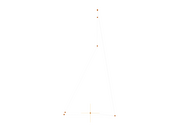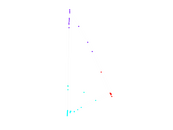### Delaunay graph drawing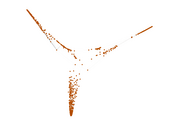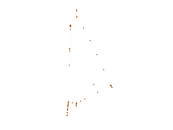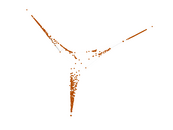### Edge weight/multiplicity distribution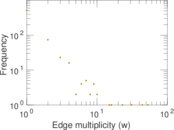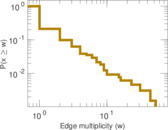### Temporal distribution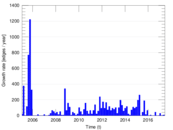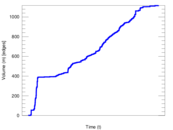### Temporal hop distribution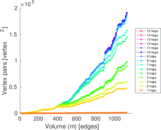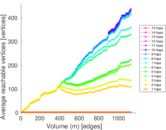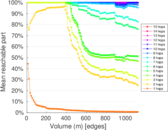### Diameter/density evolution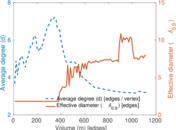### Matrix decompositions plots